# Puzzle | Maximum pieces that can be cut from a Circle using 6 straight lines

• Difficulty Level : Medium
• Last Updated : 03 Nov, 2020

PUZZLE:

Given a Circle and Six Straight Lines. What is the maximum number of pieces that one can cut the circle using these six straight lines?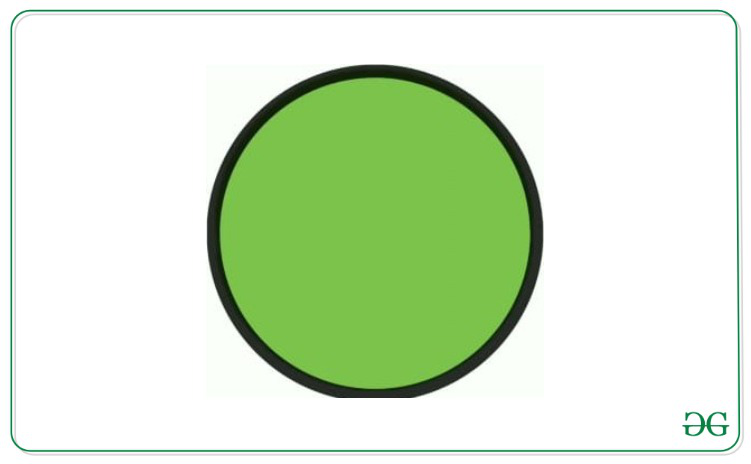SOLUTION:

Using six lines, we can cut the circle into 22 pieces at maximum.

• Using one line, the circle can be divided into two parts.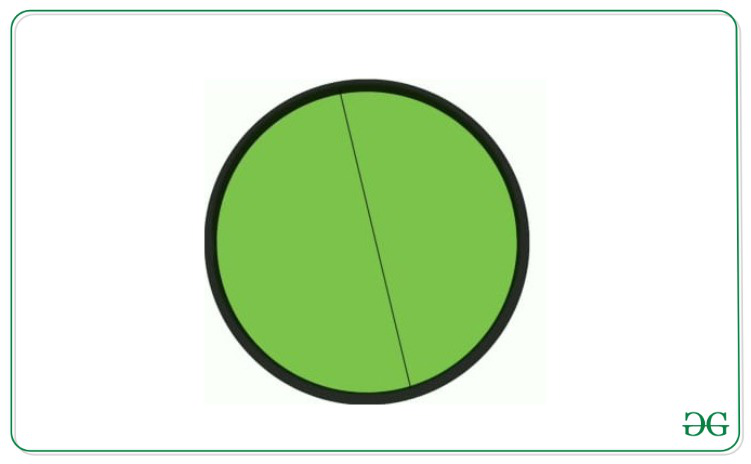• Two lines divide the circle into four parts.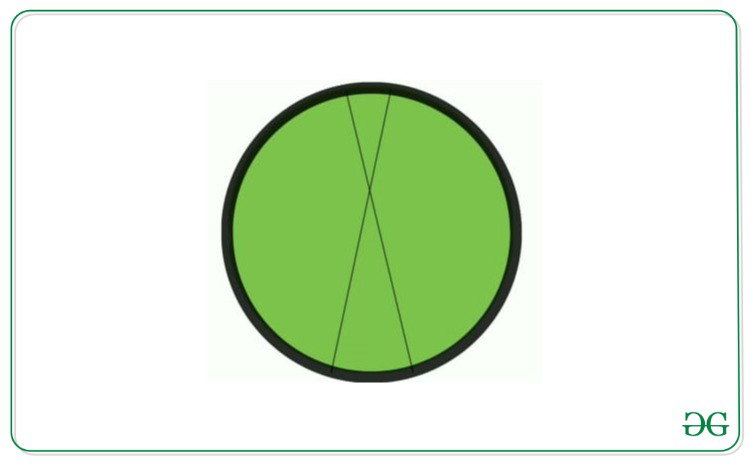• Three lines divide the circle into seven unequal parts.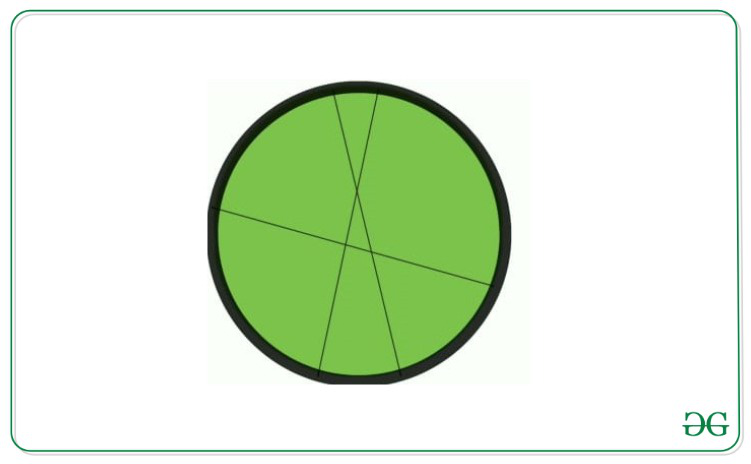• Four lines cut the circle into eleven parts.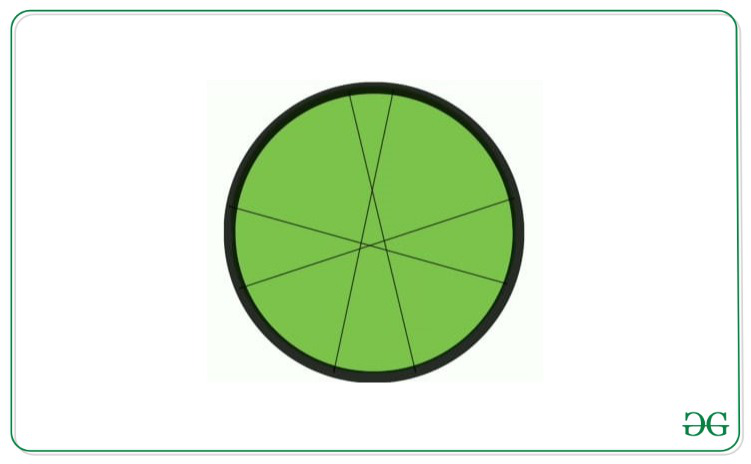• Using five lines, circle can be divided into sixteen parts.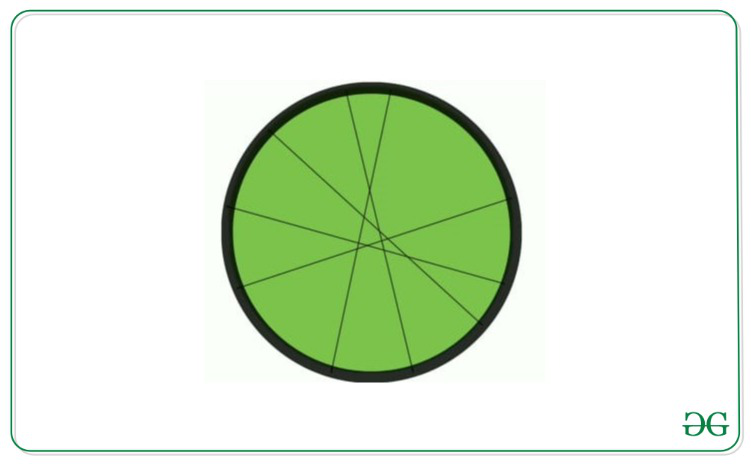• Finally, using six lines the maximum parts that can be formed are 22.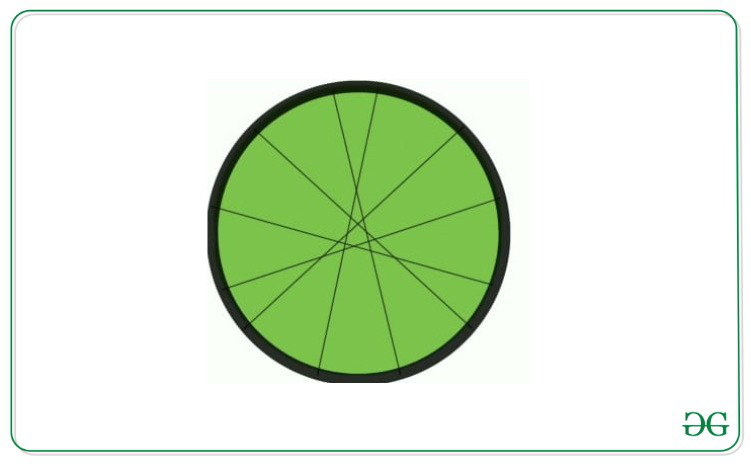My Personal Notes arrow_drop_up